Monday, August 26, 2019
Home > Latest Announcement > JSTSE 2018-19 Question Paper With Answer Keys and Solutions

# JSTSE 2018-19 Question Paper With Answer Keys and Solutions

Download This JSTSE 2018-19 Answer Keys With Solutions As PDF. Link is at Last

# JSTSE 2018-19 Question Paper With Answer Keys And Solutions

Hi students, welcome to AmansMathsBlogs (AMB). On this article, you will get JSTSE 2018-19 Question Paper With Answer Keys And Solutions.

JSTSE 2018-19 is an exam that is conducted by science branch of the Directorate of Education, Government of Delhi. In 2018-19 session, JSTSE is conducted on 20 January 2019 (Sunday).

The math questions of JSTSE 2018-19 Question Paper are solved by me, AMAN RAJ, I am maths faculty of IIT Maths Foundation course and founder of this website AmansMathsBlogs (AMB).

## JSTSE 2018-19 Answer Keys: PART – I

General Knowledge JSTSE 2018-19 Answer Keys
Ques No Ans Key Ques No Ans Key Ques No Ans Key Ques No Ans Key
1 1 16 3 31 4 46 2
2 4 17 4 32 3 47 4
3 3 18 3 33 1 48 4
4 1 19 4 34 1 49 2
5 1 20 1 35 2 50 4
6 3 21 2 36 4
7 4 22 2 37 2
8 2 23 3 38 2
9 1 24 2 39 2
10 1 25 2 40 2
11 1 26 1 41 3
12 2 27 2 42 4
13 4 28 1 43 3
14 1 29 4 44 3
15 3 30 1 45 4

## JSTSE 2018-19 Answer Keys: PART – II

General Science (51 to 170) & Mathematics (171 to 200) JSTSE 2018-19 Answer Keys
Ques No Ans Key Ques No Ans Key Ques No Ans Key Ques No Ans Key
51 1 91 2 131 3 171 2
52 4 92 1 132 1 172 3
53 1 93 4 133 2 173 4
54 2 94 1 134 2 174 2
55 2 95 2 135 1 175 3
56 2 96 2 136 1 176 3
57 2 97 1 137 3 177 1
58 1 98 4 138 2 178 2
59 1 99 2 139 1 179 1
60 1 100 2 140 2 180 2
61 2 101 4 141 4 181 1
62 3 102 4 142 3 182 2
63 2 103 3 143 4 183 4
64 1 104 3 144 2 184 4
65 3 105 1 145 3 185 2
66 2 106 1 146 2 186 4
67 3 107 4 147 2 187 4
68 2 108 3 148 3 188 1
69 3 109 3 149 4 189 1
70 2 110 3 150 2 190 3
71 3 111 2 151 1 191 2
72 1, 2 112 3 152 1 192 3
73 2 113 2 153 3 193 1
74 2 114 4 154 1 194 4
75 4 115 1 155 4 195 3
76 3 116 2 156 4 196 2
77 2 117 4 157 2 197 2
78 2 118 2 158 1 198 3
79 3 119 4 159 3 199 2
80 3 120 1 160 4 200 3
81 4 121 3 161 2
82 2 122 2 162 1
83 2 123 4 163 3
84 2 124 2 164 4
85 4 125 1 165 3
86 3 126 3 166 4
87 2 127 3 167 1
88 1 128 1 168 2
89 3 129 3 169 2
90 1 130 4 170 3

## JSTSE 2018-19 Answer Keys With Solutions (Math)

JSTSE 2018-19 Question Paper With Solutions: Ques No 171

If x =, then the value of x2 + (39/x)2 is

Options:

A. 104

B. 114

C. 124

D. 144

Answer Key: B

Solution: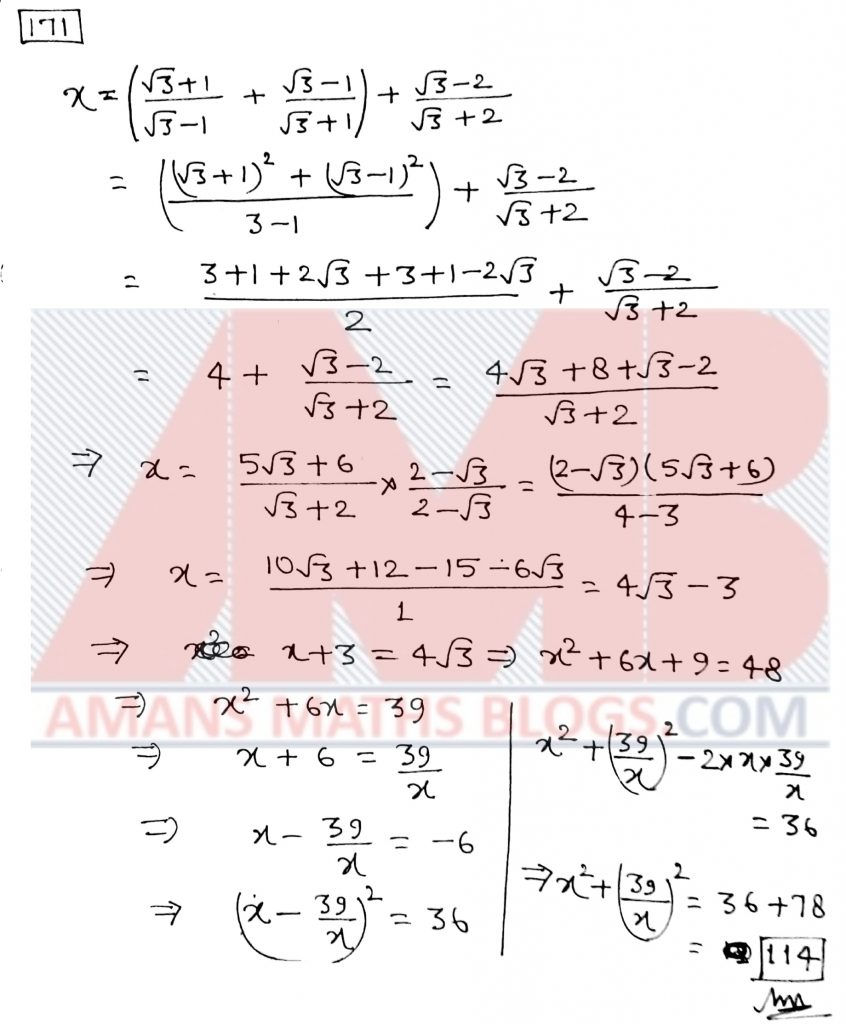JSTSE 2018-19 Question Paper With Solutions: Ques No 172

If a/b + b/a = 1, (a, b0) then the value of a3 + b3 is

Options:

A. 1

B. -1

C. 0

D. 2

Answer Key: C

Solution:JSTSE 2018-19 Question Paper With Solutions: Ques No 173

If value ofis

Options:

A. 8(3)^(1/2)

B. 2(3)^(1/2)

C. 3(2)^(1/2)

D. (6)^(1/2)

Answer Key: D

Solution:JSTSE 2018-19 Question Paper With Solutions: Ques No 174

If one angle of a triangle is 130, then the angle between the angle bisectors of the other two angles is

Options:

A. 145 degree

B. 155 degree

C. 135 degree

D. 95 degree

Answer Key: B

Solution: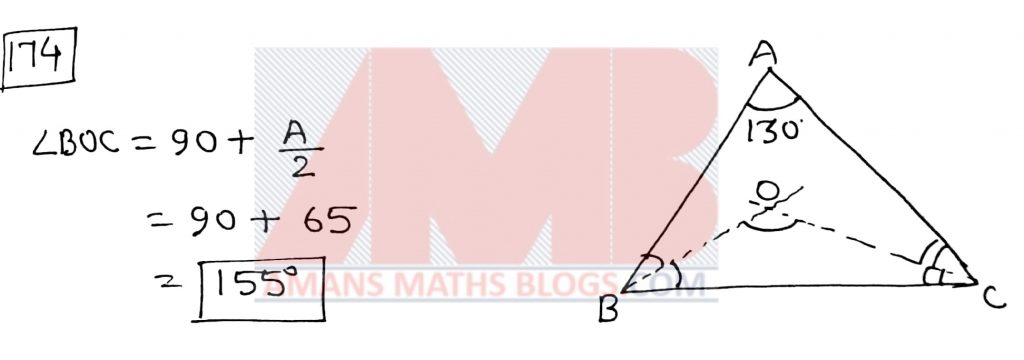JSTSE 2018-19 Question Paper With Solutions: Ques No 175

If 4x – 4x-1 = 24, then (2x)x equals

Options:

A. 5Root(5)

B. Root(5)

C. 25Root(5)

D. 125

Answer Key: C

Solution: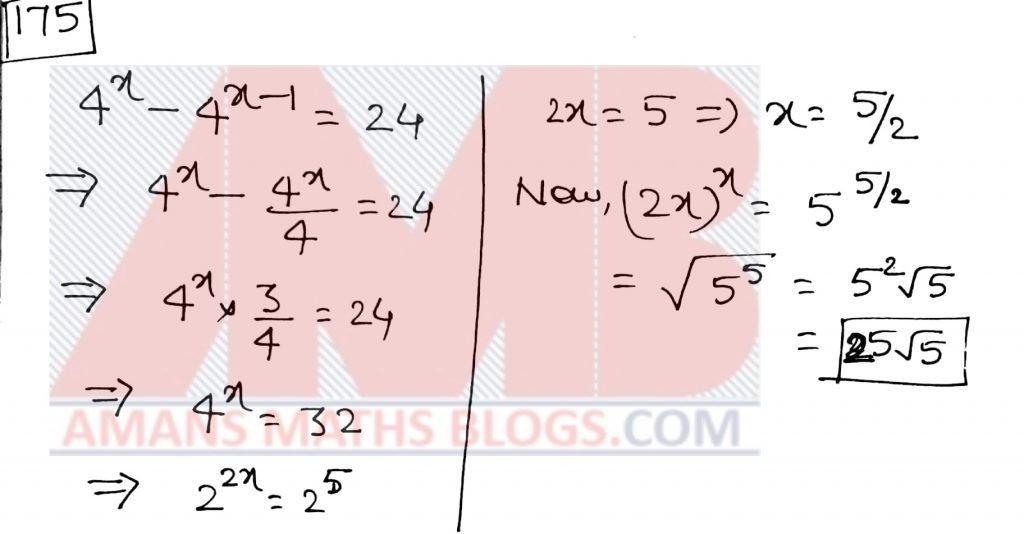JSTSE 2018-19 Question Paper With Solutions: Ques No 176

If [(2.3)3 – (0.027)]/[(2.3)2 + 0.69 + 0.09] = k, then the value of k is

Options:

A. 2.273

B. 2.327

C. 2

D. 3

Answer Key: C

Solution: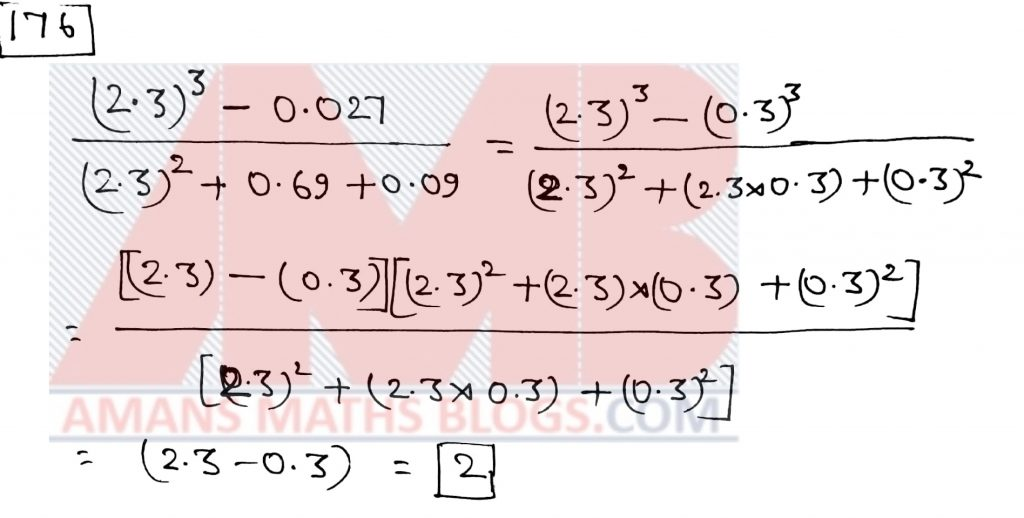JSTSE 2018-19 Question Paper With Solutions: Ques No 177

If (3x – 1)7 = a7x7 + a6x6 + a5x5 + … + a1x + a0, then the value of (a7 + a6 + a5 + a4 + a3 + a2 + a1 + a0) is

Options:

A. 128

B. 64

C. 0

D. 32

Answer Key: A

Solution:JSTSE 2018-19 Question Paper With Solutions: Ques No 178

If a : b = 2 : 3, x : y = 3 : 4, then the value of (25by – 2ax)/(3ay + 4bx) is

Options:

A. 5 : 24

B. 24 : 5

C. 1 : 2

D. 2 : 1

Answer Key: B

Solution:JSTSE 2018-19 Question Paper With Solutions: Ques No 179

If x3 – 3x2 + 3x + 7 = (x + 1)(ax2 + bx + c), then the value of (a + b + c) is

Options:

A. 4

B. 12

C. -10

D. 3

Answer Key: A

JSTSE 2018-19 Question Paper With Solutions: Ques No 180

In the given figure (see QNo: 180 in above question paper), PQRS is a square. RST is an equilateral triangle, then the value of x is.

Options:

A. 60 degree

B. 75 degree

C. 45 degree

D. 80 degree

Answer Key: B

Solution:JSTSE 2018-19 Question Paper With Solutions: Ques No 181

One angle of a pentagon is 140 degree. If the remaining angles are in the ratio of 1:2:3:4, then the greatest angle is

Options:

A. 160 degree

B. 170 degree

C. 140 degree

D. 150 degree

Answer Key: A

Solution:JSTSE 2018-19 Question Paper With Solutions: Ques No 182

If the mean of age of 18 students of a class is 14.5 years, two more students of ages 15 years and 16 years join the class, then the new mean of age is

Options:

A. 14 years

B. 14.6 years

C. 14.5 years

D. 14.7 years

Answer Key: B

Solution:JSTSE 2018-19 Question Paper With Solutions: Ques No 183

The value ofis

Options:

A. 9

B. 8

C. 6

D. 7

Answer Key: D

Solution: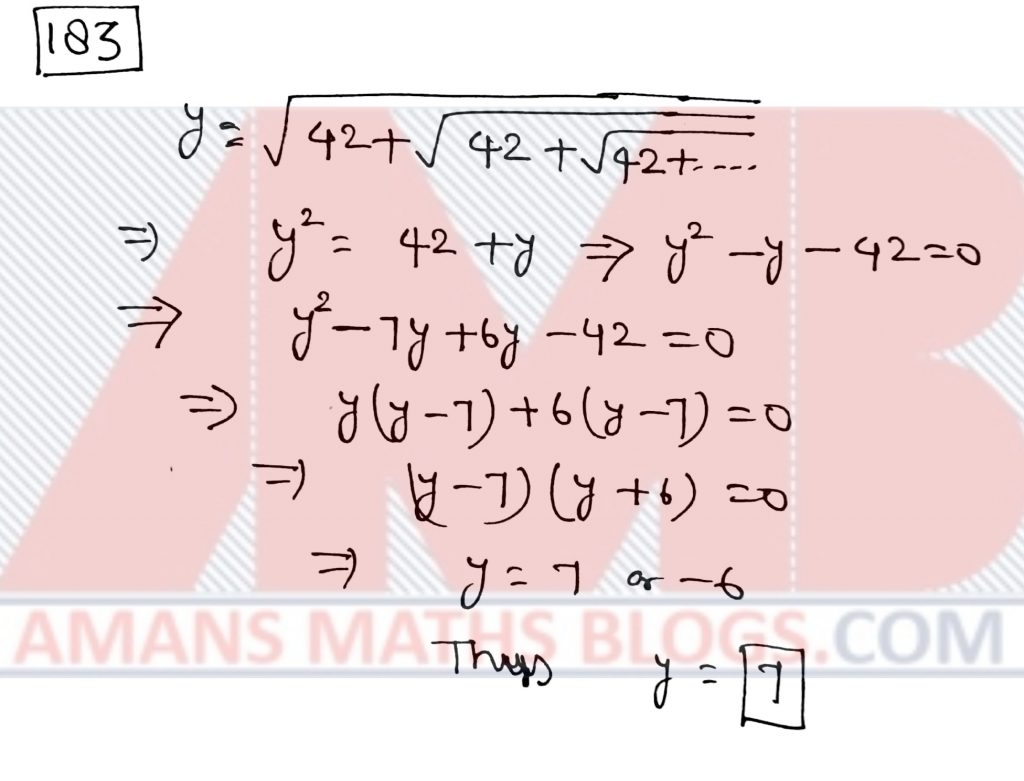JSTSE 2018-19 Question Paper With Solutions: Ques No 184

In the given triangle RST (see QNo: 184 in above question paper), the value of x is

Options:

A. 40 degree

B. 90 degree

C. 80 degree

D. 100 degree

Answer Key: D

Solution: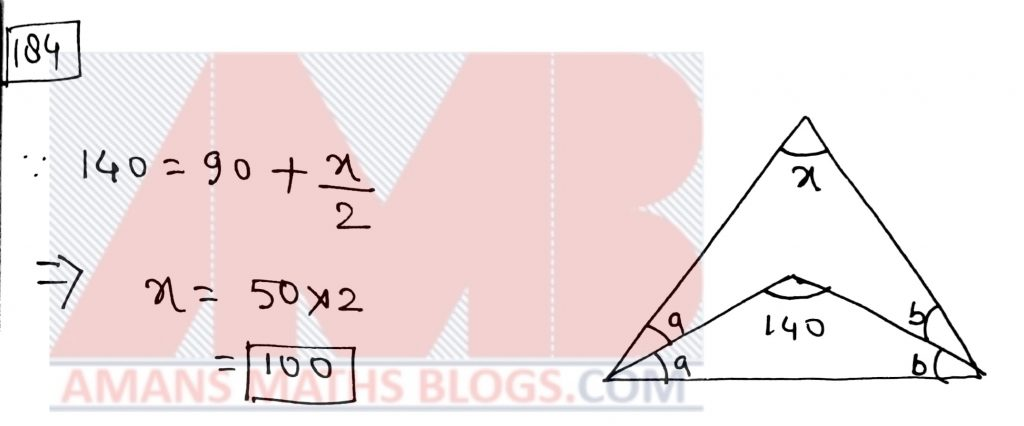JSTSE 2018-19 Question Paper With Solutions: Ques No 185

If the cost price of 25 articles is equal to the selling price of 20 article, the gain percentage is

Options:

A. 20%

B. 25%

C. 5%

D. 10%

Answer Key: B

Solution:JSTSE 2018-19 Question Paper With Solutions: Ques No 186

The value ofis

Options:

A. 3/22

B. 41/100

C. 1/2

D. 9/22

Answer Key: D

Solution: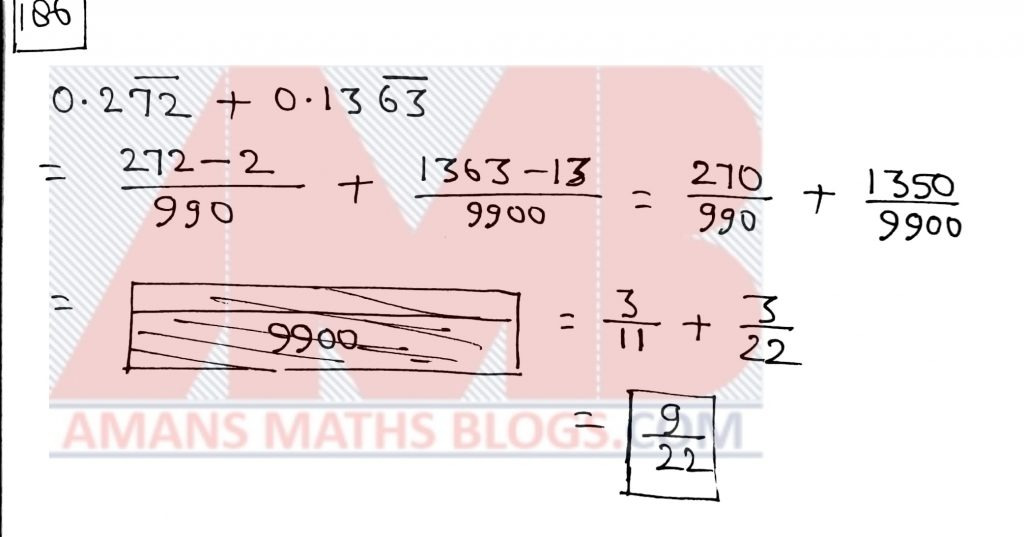JSTSE 2018-19 Question Paper With Solutions: Ques No 187

If a = 2 + Root(3), then the value of (a – 1/a) is

Options:

A. 0

B. 2 + Root(3)

C. 4

D. 2Root(3)

Answer Key: D

Solution:JSTSE 2018-19 Question Paper With Solutions: Ques No 188

In the given figure (see QNo: 188 in above question paper), AB || HG, then the value of a is

Options:

A. 8

B. 9

C. 7.5

D. 10

Answer Key: A

Solution: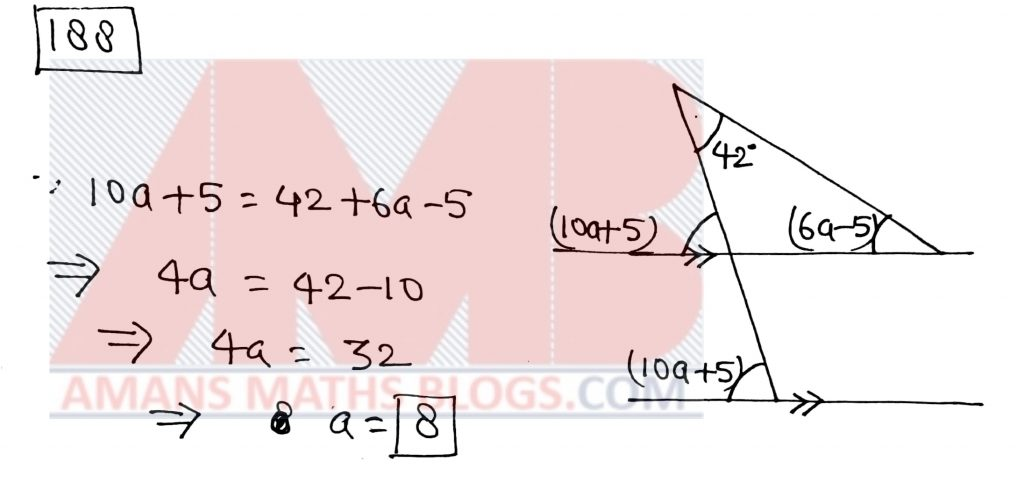JSTSE 2018-19 Question Paper With Solutions: Ques No 189

If one factor of (x4 + x2 – 20) is (x2 + 5). The other factor is

Options:

A. x2 – 4

B. x2 + 4

C. x – 4

D. x + 20

Answer Key: A

Solution:JSTSE 2018-19 Question Paper With Solutions: Ques No 190

If x = 7 + 4Root(3) and xy = 1, then (1/x2 + 1/y2) is

Options:

A. 64

B. 134

C. 194

D. 149

Answer Key: C

Solution: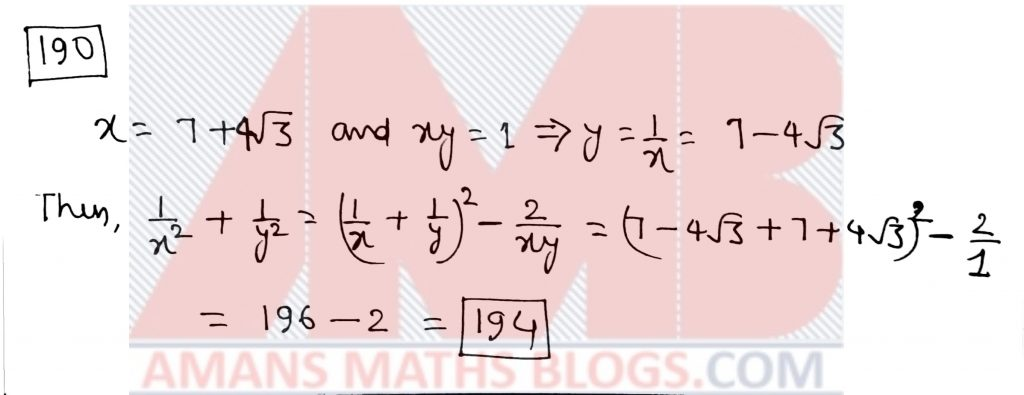JSTSE 2018-19 Question Paper With Solutions: Ques No 191

If 52m-1 = 25m-1 + 100, then the value of 32+m is

Options:

A. 27

B. 81

C. 9

D. 343

Answer Key: B

Solution:JSTSE 2018-19 Question Paper With Solutions: Ques No 192

If 5 coins are tossed together, what will be the probability of getting at least two heads?

Options:

A. 1/32

B. 3/16

C. 13/16

D. 5/16

Answer Key: C

Solution:JSTSE 2018-19 Question Paper With Solutions: Ques No 193

If, then the value of (x – y) is

Options:

A. 16

B. 38

C. 22

D. 21

Answer Key: A

Solution: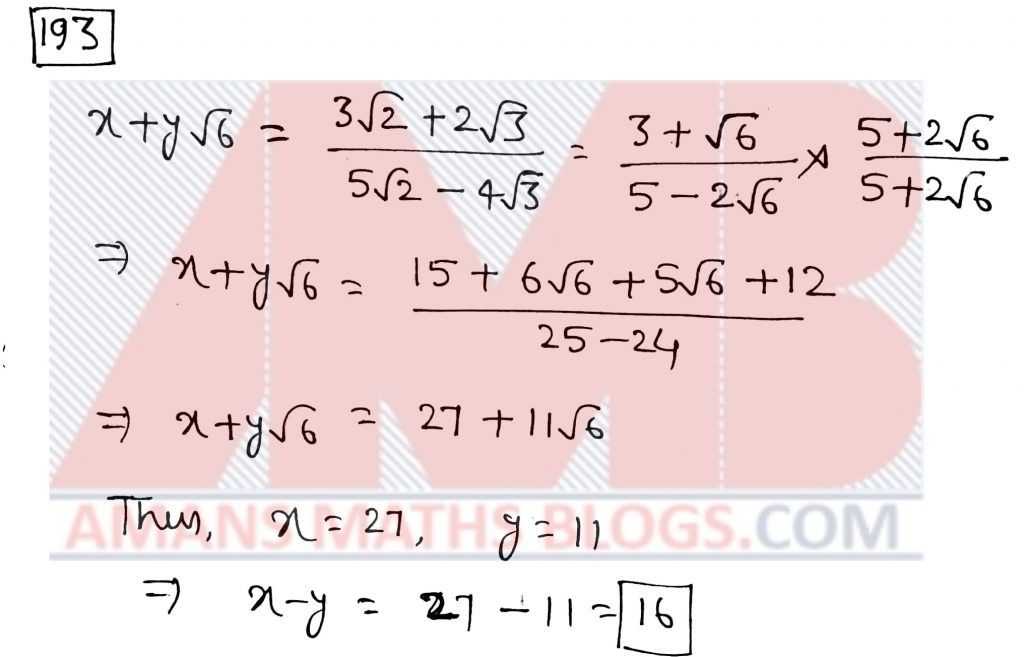JSTSE 2018-19 Question Paper With Solutions: Ques No 194

If p + q + r = 0, then the value of (q + r)2/qr + (r + p)2/rp + (p + q)2/pq is

Options:

A. 0

B. 1

C. 2

D. 3

Answer Key: D

Solution: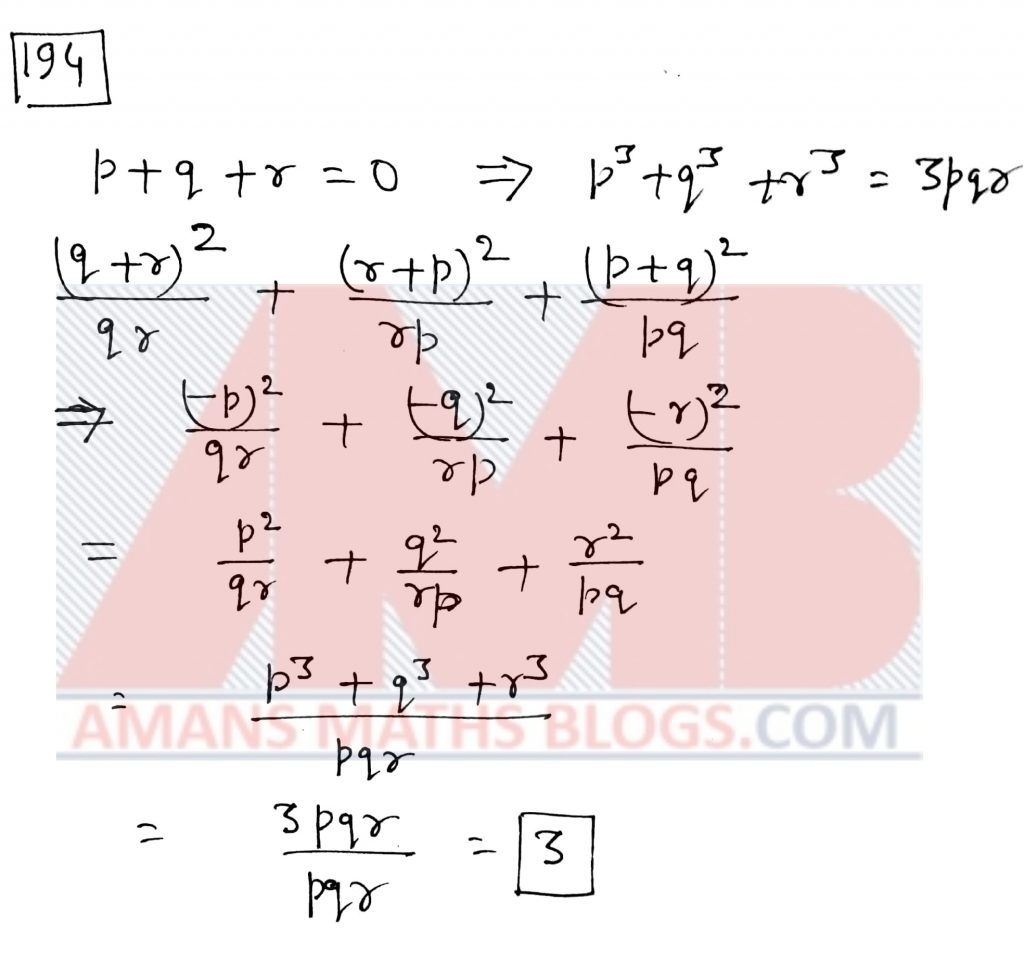JSTSE 2018-19 Question Paper With Solutions: Ques No 195

If 2l – m + n = 0, then the value of 4l2 – m2 + n2 + 4ln is

Options:

A. 1

B. -1

C. 0

D. 2

Answer Key: C

Solution: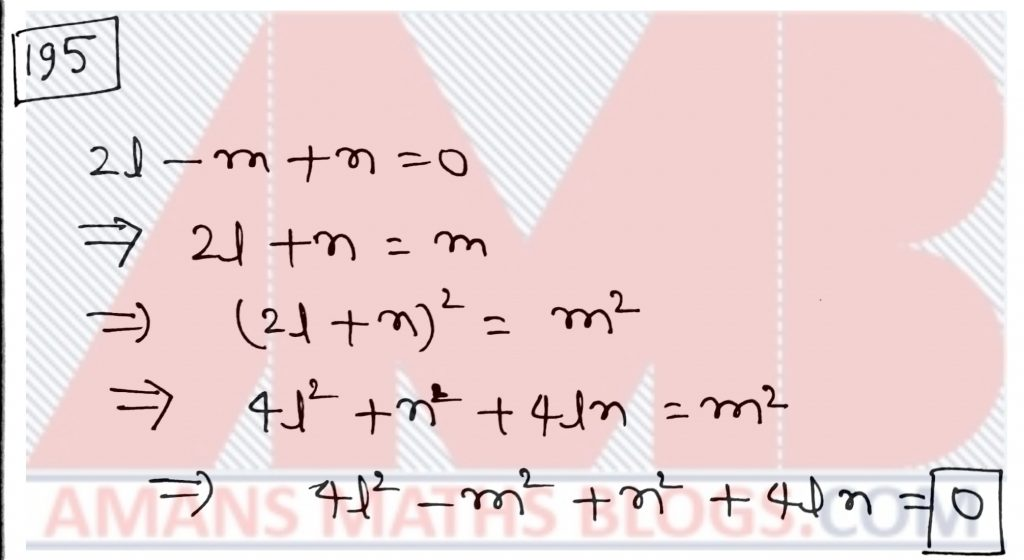JSTSE 2018-19 Question Paper With Solutions: Ques No 196

If m2 + 1/25m2 = 43/5, then the value of m3 + 1/125m3 is

Options:

A. 50(1/5)

B. 25(1/5)

C. 12(1/5)

D. 15(1/5)

Answer Key: B

Solution:JSTSE 2018-19 Question Paper With Solutions: Ques No 197

The cost price of a bag and a book is Rs. 371. If the bag costs 12% more than the book, then the cost price of the bag is

Options:

A. Rs. 175

B. Rs. 196

C. Rs. 150

D. Rs. 221

Answer Key: B

Solution: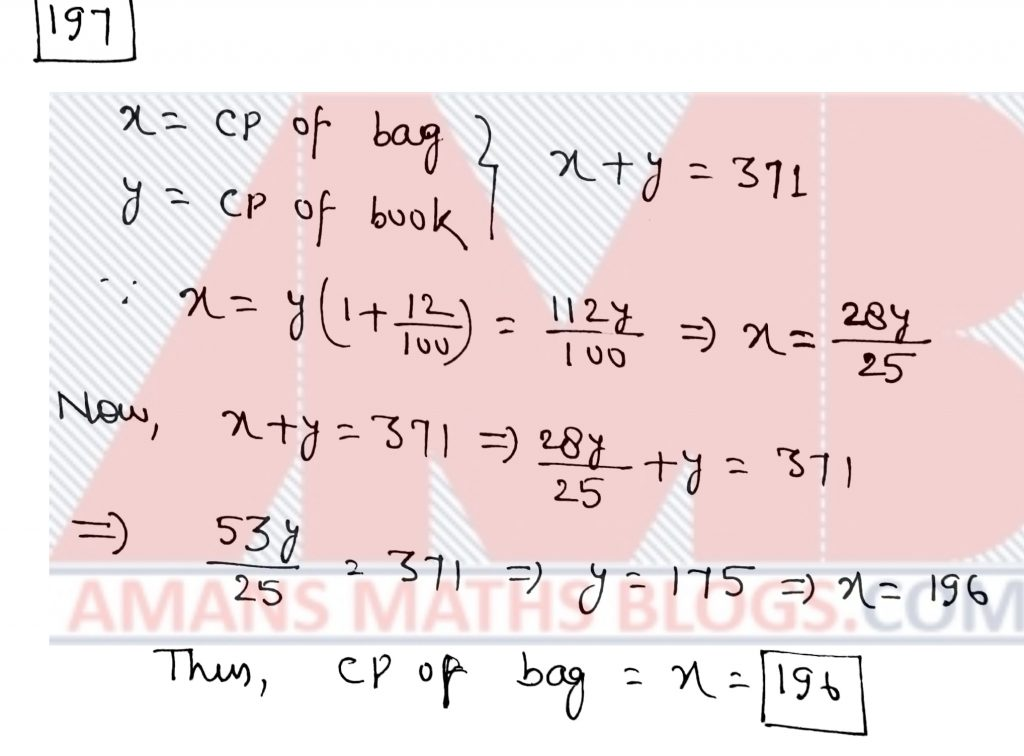JSTSE 2018-19 Question Paper With Solutions: Ques No 198

In the given figure (see QNo: 198 in above question paper), AE = DC = 13 cm, BE = 5 cm, angle ABC = 90 degree and AD = EC, then the length of AD is

Options:

A. 5 cm

B. 6 cm

C. 7 cm

D. 12 cm

Answer Key: C

Solution: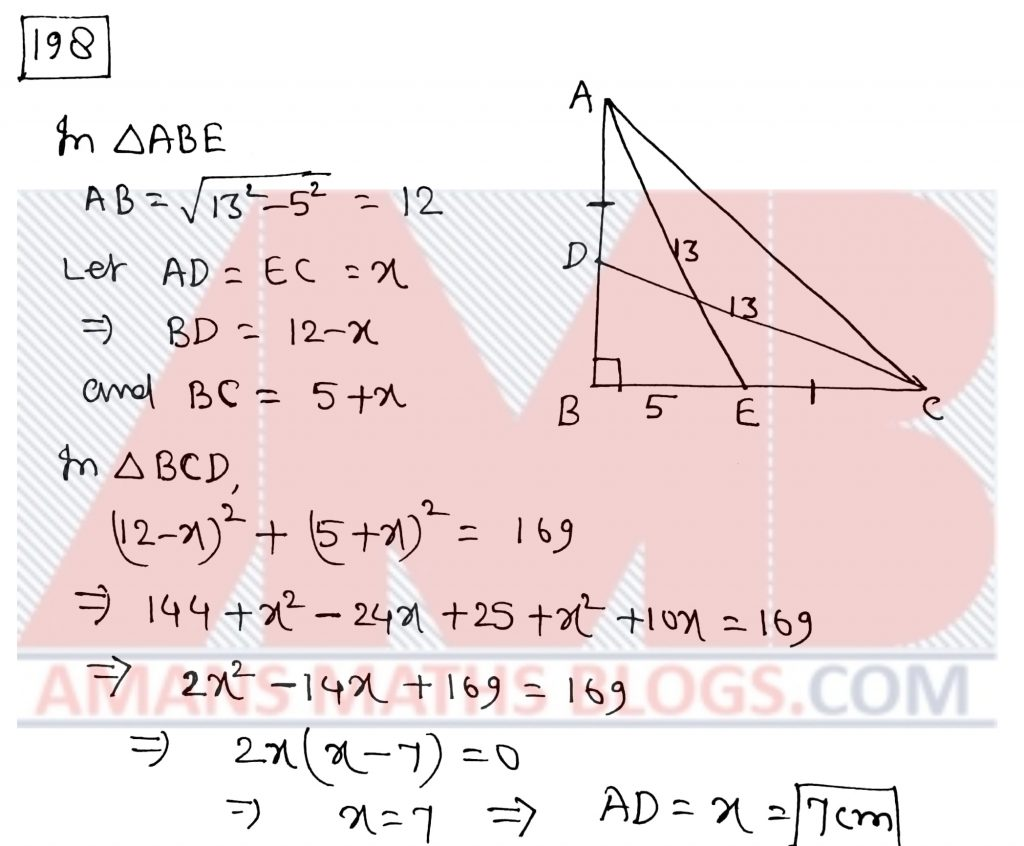JSTSE 2018-19 Question Paper With Solutions: Ques No 199

Two dice are rolled, then the probability of getting a total of 9 is

Options:

A. 1/3

B. 1/9

C. 9/10

D. 8/9

Answer Key: B

Solution: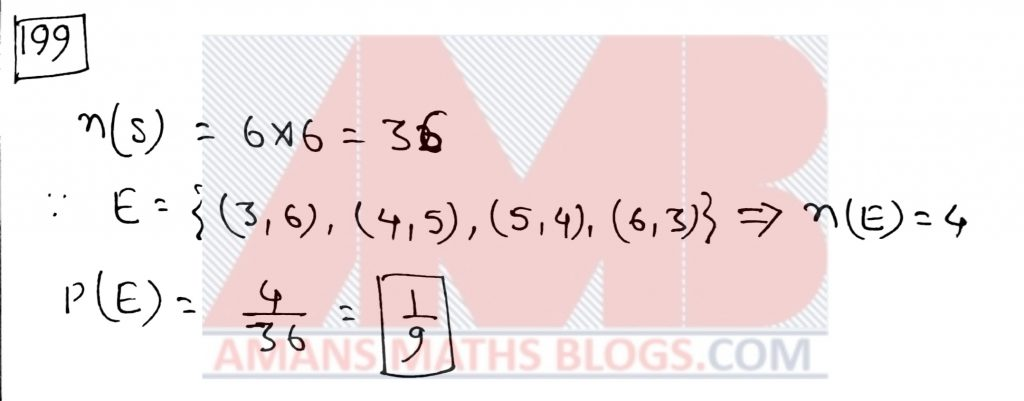JSTSE 2018-19 Question Paper With Solutions: Ques No 200

If A:B:C = 2:3:4 and A2 + B2 + C2 = 11600, then the value of (A + B + C) is

Options:

A. 20

B. 24

C. 60

D. 100

Answer Key: C

Solution:Click Here To Download This As PDF

error: Content is protected !!